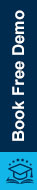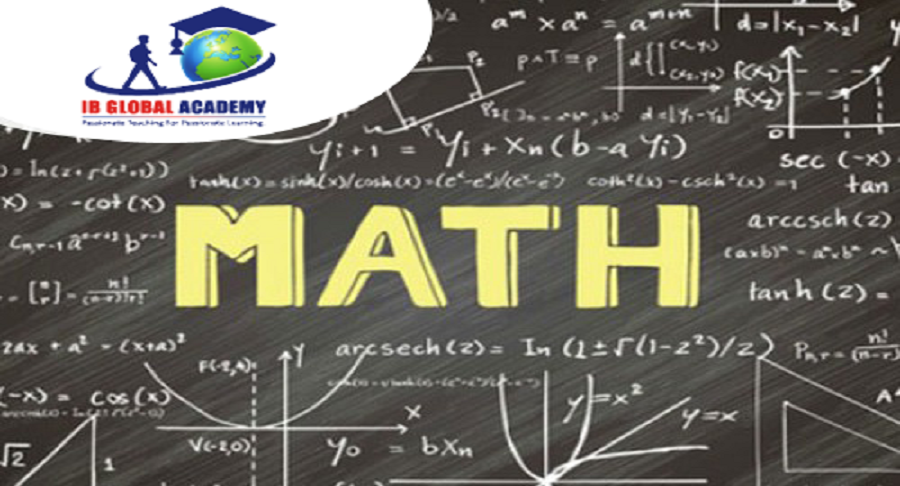# Calculus: Applications and Beyond in the view of an IB maths tutor09/13/2023

Calculus is a subject that is studied extensively in the IB mathematics curriculum. It is a potent instrument that makes it possible for us to comprehend and examine intricate mathematical processes.

Sir Isaac Newton, a British scientist, created this new branch of mathematics between 1642 and 1727. The Latin term for "calculus" is "stone." Romans counted using stones. Mathematicians started using the same word for counting infinitely tiny numbers.

Even though it was shown that ancient Indian mathematicians were familiar with certain calculus fundamentals, Newton & Leibnitz heralded a new age in mathematics. Calculus is used in a wide variety of ways in everyday life. It cannot possibly be shown in just one view. Here, I'm attempting a brief description of them.

The intriguing realm of calculus will be explored in-depth in this blog article, moving beyond the IB syllabus to look at its applications. This blog article will provide you with insightful knowledge and fascinating notions about calculus, whether you are an IB learner or just interested in mathematics- and help can be taken from an IB maths tutor in Gurgaon-India!

Calculus gives us a strong mathematical foundation for analysing complicated processes, comprehending rates of change, and addressing real-world issues.

We may better grasp and appreciate the aesthetic value of calculus by exploring the ideas and applications outside of the IB syllabus. So let's accept the challenge and solve the maths riddles together!

Real world and Calculus

In many disciplines, such as physics, engineering, economics, and computer science, calculus is an essential tool. Calculus is essential for modeling and comprehending the behavior of physical systems in physics. In fields like mechanics, thermodynamics, electromagnetism, and quantum mechanics, it is often utilized to calculate motion, forces, and energy.

Calculus offers resources for studying rates of change, optimization, and equilibrium in economics. It is employed in the study of ideas like utility maximization, cost and manufacturing functions as well as supply and demand. Calculus aids economists in their modelling, forecasting, and decision-making.

Incorporated into Medical Science

• Differential calculus is a tool used by biologists to calculate the precise rate of development in a bacterial culture when several factors, such as food supply and temperature, are modified.

Physics application

• Integration is essential in physics. For instance, to determine a sports utility vehicle's Centre of Gravity, Centre of Mass, and Mass Moment of Inertia.
• To determine an object's speed and trajectory, forecast the positions of planets, and comprehend electromagnetism.

Implementation in Engineering

• In order to calculate the weight of curved form structures (such as the dome above a sports stadium) and the amount of materials required to build them, an architect-engineer employs integration.
• Not only are buildings but also crucial infrastructures like bridges benefit from the application of calculus in design.
• Calculus (Integration) in Electrical Engineering is used to calculate the precise length of the power line required to connect 2 substations that are located miles apart from one another.
• When making long-term plans, space flight engineers commonly employ calculus. They must take into account other gravitational factors, like as the sun while launching an exploratory probe, as well as the various orbital velocities of the planet and the Earth it is intended to visit.

Construction Industrially

The architect is a good illustration of someone who uses mathematics in the workplace. To translate design drawings onto printed representations that construction workers may use as models for actual structures, architects employ calculus.

Architects use calculus to analyze and calculate structural issues in order to come up with a solution that ensures the structure will stand firm and stable.

Without mathematics, it would not be able to create a print like that to depict the architect's design. And with the illustration of the use of calculus in the workplace, maybe it will motivate us to study calculus more industriously in the future.

Tips to prepare calculus

It is important to understand that the time efficient strategy to study is important not only for calculus, but it is effective for other subjects also.

You'll develop into a more assured, prosperous, and well-rounded learner as a result of this. Your study and amusement time will be perfectly balanced. You can also consider taking guidance from an IB tutor in India from IBGA.

One must spend at least couple of hours over the calculus assignments daily. This gives you a longer period to focus on difficult homework assignments and aids in the organization of your questions, concerns, and ideas.

Your ability to ask your classmates and professors questions that are both clear and succinct increases as you spend more time on your assignments.

Calculus must be practiced daily because you can become more used to ideas, definitions, and theorems by practicing calculus every day. Calculus will become more and simpler as a result of this familiarity.

Start working on new problems with an intention to prepare for exam. And the text book, practice exercise and past papers are the best approach to prepare well for exams. Good luck for exams!